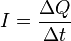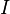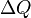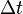# Electric current facts for kids

Kids Encyclopedia Facts

An electric current is a flow of electric charge. The equation of current is:$I = \frac{\Delta Q}{\Delta t}$
where$I$ is the current flowing$\Delta Q$ is the change in electric charge$\Delta t$ is the change in time

The SI unit of electric current is the ampere (A). This is equal to one coulomb of charge in one second. Current can be found in wires, batteries, and lightning.

## The source of current

In conducting materials, some electrons are very loosely bound to the atoms of the material. When large amounts of these atoms come together, there is a sort of electron cloud that "hovers" near the atoms of the material. If you examine a cross-section of the piece of conducting material, the electrons will move very quickly through it. This motion is caused by temperature, and electrons flowing in one direction tend to equal the electrons flowing from the other direction, so this is not what causes current to flow. Electrons flow from one atom to another, a process has been compared to the passing of water buckets from one person to another in a bucket brigade.

When an electric field is put on the wire, the electrons respond almost instantly by drifting slightly in the opposite direction of the field. They gain energy from the field, which is lost very quickly when they bump into other electrons in the material. As long as the field is in place, however, the electrons will gain back that energy that they lost, and the process will continue. This "jolt" that electrons receive from the electric field is the source of current, not the overall flow of electrons themselves. From this discussion, we can see two things that current is not:

• It is not an actual "flow" of electrons in the everyday sense of the word: If we examine the speed given to the electrons by the field, it is usually very small, on the order of millimeters per second. It would take half an hour for electrons to cross a 10-foot (3 m) room at this rate. Since a light bulb flips on almost immediately after hitting the switch, something else must be at work.
• It is also not a "domino effect", although this analogy is closer than the flow. Since electrons are so tiny, even when they are moving very quickly they do not have a lot of force behind them.

## Current in circuits

When current is flowing in a wire circuit, it accelerates when there is no resistance in the circuit. Resistors are used to increase resistance in the circuit so it slows down the current. The relationship between resistance, current, and voltage (another part of the circuit) is shown by Ohm's law.Electric current Facts for Kids. Kiddle Encyclopedia.